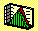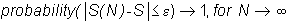2.5 SAMPLE STATISTICS: CONSISTENCY

When parameters of a population are estimated from a random sample drawn from the population, the larger the sample is, the better your estimate of the population parameter should be. The statistical concept of consistency formalizes this intuition.

Let S(N) be an estimate of some population parameter S, given N points in the sample, and let e be a positive real number. The sample estimate is a consistent estimate if for any e:This definition requires that a sample statistic converges in probability to the population statistic. In this case, the probability that the distance between the statistic and the population parameter is less than any number approaches one as the sample size becomes large.

Both the sample mean and variance (or standard deviation) of a sample that has been randomly drawn from the entire population, satisfy the property of consistency. In the next topic we consider the property, unbiasedness.

previous topic

next topic